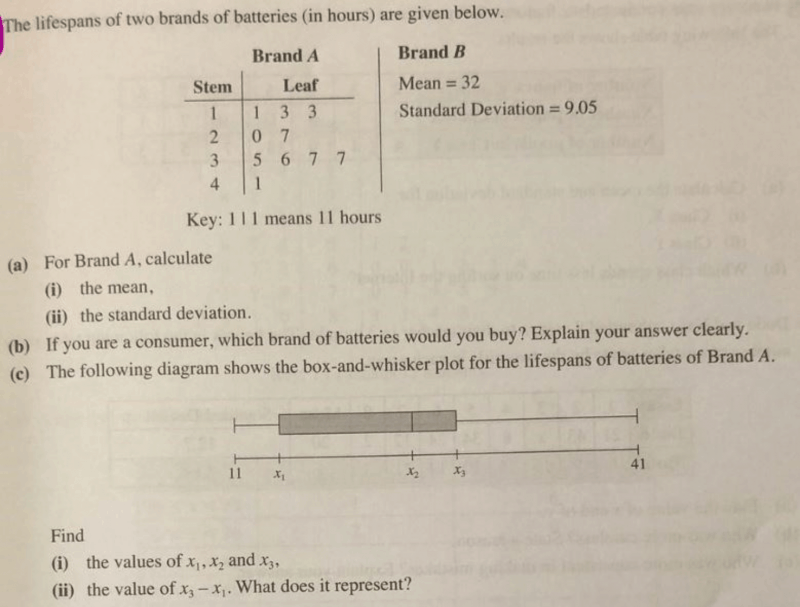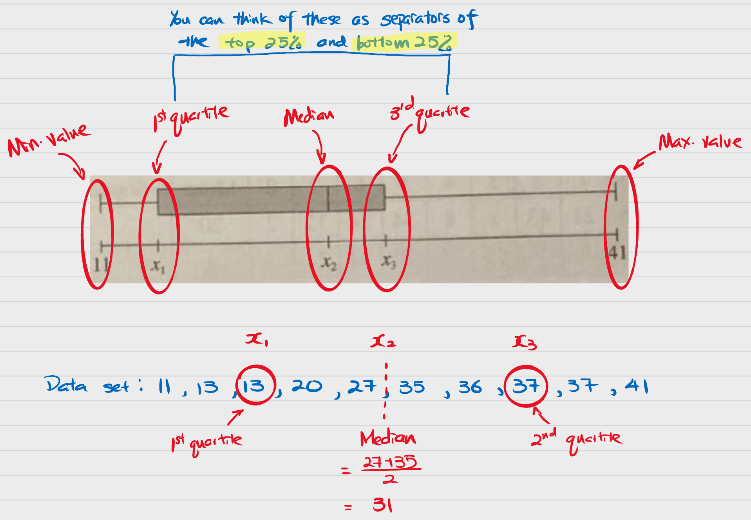# QuestionHi, can help with qn c pls?The short answer is that that is how the box and whisker plot is to be read. The box and whisker plot is designed to give people a a quick five-point summary of the data set:

1. Minimum value
2. 1st quartile (top 25% or basically the median between the median of the whole data set and the minimum value)
3. Median
4. 3rd quartile (bottom 25% or basically the median between the median of the whole data set and the maximum value)
5. Maximum value
6. Outliers (extreme values if they are really significant)

0 Replies 1 Like ✔Accepted Answer

x2 is the median. x2 = (27+35)/2= 31

x1 is the first quartile, which is the third value here = 13

x3 is the third quartile, which is the 8th value = 37

x3-x1 is 37-13=24. This is known as the interquartile range, which is the spread of the data around the median.

Do contact me (number in profile) if you need tuition!

why is the first quartile the 3rd value? and the third quartile the 8th value?